# Generating a PSD

June 25, 2019

Vibration Research software uses Welch’s method for PSD estimation. The process begins with Gaussian-distributed time-domain input data (a time history file).

Here is a summarization of the steps to generate a PSD:

• Data is partitioned into frames of equal length in time; each frame is transformed into the frequency domain using the Fast Fourier Transform (FFT)
• Frequency-domain data is converted to power by taking the squared-magnitude of each frequency point; the squared-magnitudes (power values) for each frame are averaged
• The average is divided by the sample rate to normalize to a single hertz

A few additional points—windowing and overlapping specifically—also influence the overall result. Let’s look at each step in more detail.

### How to Generate a PSD

Figure 1.1 shows five seconds of Gaussian-distributed random vibration data in a time history file (Acceleration vs. Time). It is difficult to extract any meaningful information from the graph other than the peak acceleration, which appears to be around -30G.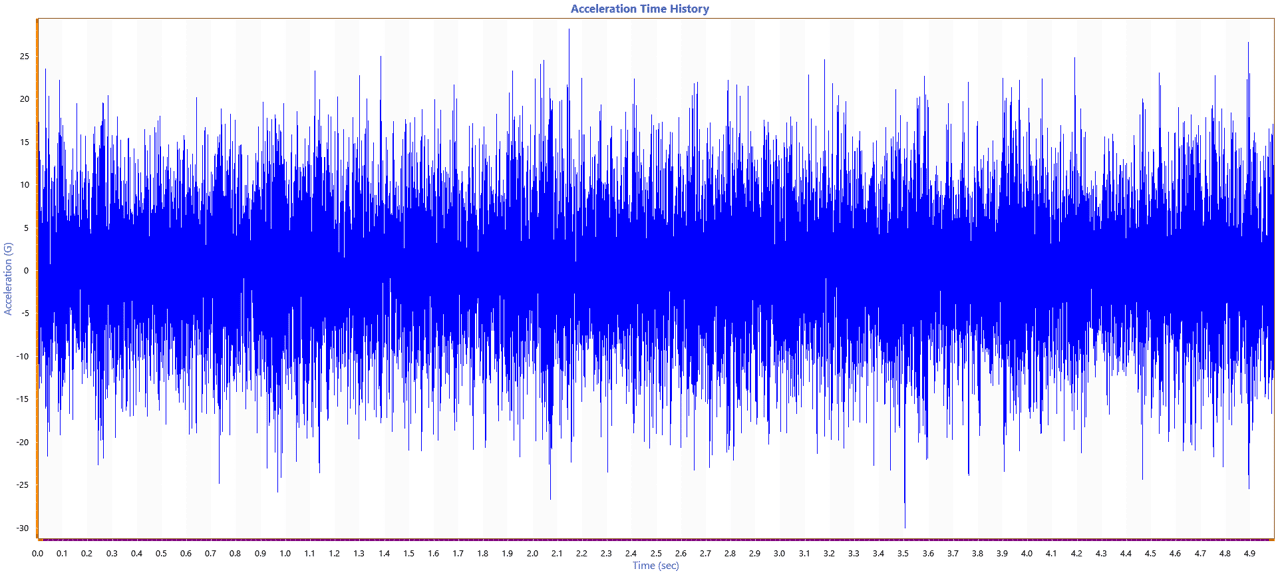Figure 1.1. A five-second time history graph.

To extract useful information from the vibration data, it must be viewed in the frequency domain. Moving to the frequency domain requires two calculations: the FFT and the PSD.

#### Fast Fourier Transform (FFT)

The FFT transforms the data into an Acceleration vs. Frequency graph. No windowing, averaging, or normalizing functions are used to create an FFT graph.

An FFT graph is often used to monitor the frequency spectrum. It focuses on changes in the frequency spectrum while viewing live data or playing through a time history file. However, to view energy distribution across the frequency spectrum, the PSD must also be calculated.

#### Power Spectral Density (PSD)

The steps to calculating the power spectral density are as follows:

1. Divide the time history file into frames of equal time length

First, the time history file should be divided into frames of equal time length. To do so, use lines of resolution and the sample rate to determine the width of each frame. There are two samples per analysis line.

In Figure 1.2, the recorded time history was sampled at 8,192Hz and 4,096 lines of resolution resulting in a frame width of one second. If the number of lines was changed to 1,024, the frame width would be 0.25 seconds. This is an important step to remember when initially selecting a sample rate. Using a sample rate that is an exponential of 2 (2n) usually results in a PSD with lines spaced at a convenient interval.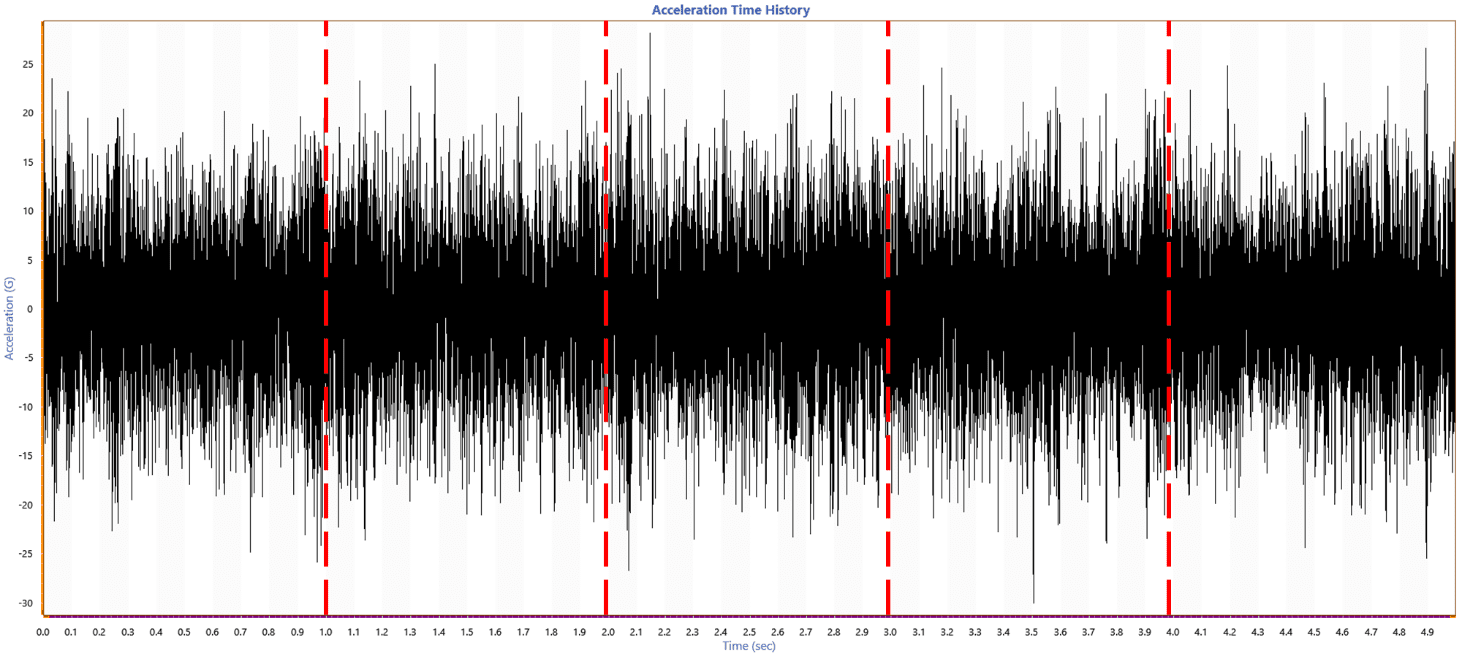Figure 1.2. The time history graph divided into frames.

2. Calculate the FTT for each frame after applying a window function

#### Applying a window function to the data

An FFT assumes data is an infinite series; therefore, the starting and ending points of each frame are interpreted as though they were next to each other. Normally, random data is not an infinite series, so a window function needs to be applied before calculating the FTT.

Without a window function, the starting and ending points may be different, resulting in a transient spike between the two points. The transient spike would show up in the FFT as high-frequency energy. Think of a terminal peak shock pulse: the sharp transition from the peak amplitude to zero acceleration generates a larger amount of high-frequency energy than a smooth pulse like the half-sine.

For the FFT, the sharp transition between starting and ending points is a discontinuity. This discontinuity is called a spectral leakage and is reflected in an FFT calculation. Applying a window function removes the emphasis on the discontinuities and reduces the spectral leakage. Ideally, the real data would have identical starting and ending points in every frame of data. As this is not the case, we must minimize any discontinuity by using a window function (Figure 1.3).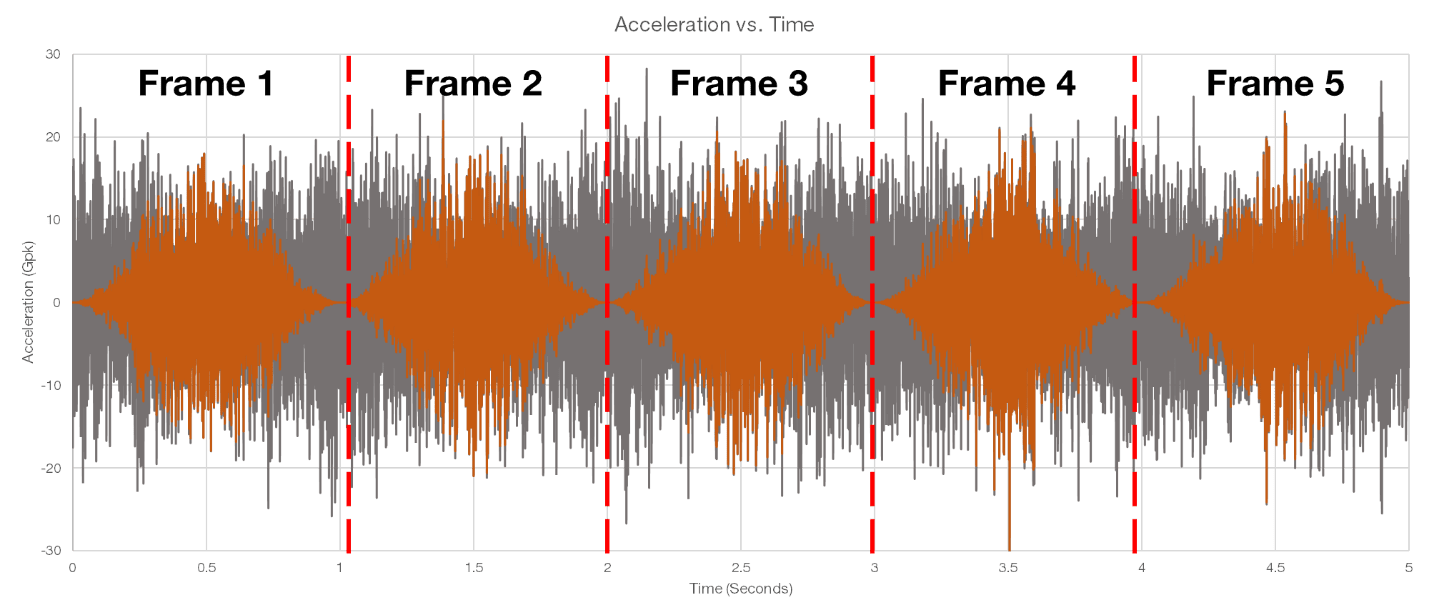Figure 1.3. Frames of data after windowing.

There is more than one window function, so we must determine which function is most suitable for the current application. A window function is evaluated by two key components: the side lobe and the main lobe. The Hanning window function is used in Figure 1.3 (the grey waveform is the original waveform and the orange waveform is the windowed data.) The Hanning function has a very high and wide main lobe and low side lobes at practically zero. There is little to no discontinuity between the starting and ending points, which results in accurate frequency measurement.

The most commonly used window functions in vibration testing are the Hanning or Blackman. Both functions have good frequency resolution, minimal discontinuity, and, therefore, minimal leakage. Other window functions may be appropriate for other applications. For a comprehensive list of commonly (and not-so-commonly) used window functions, see the VRU course Window Functions for Signal Processing.

#### Calculating the FFT for Each Frame

The windowed data is then used to calculate the FFT for each frame, transforming the signal from the time domain into the frequency domain (see Figure 1.4.) This linear transformation gives us the ability to observe the frequency content of the time history waveform.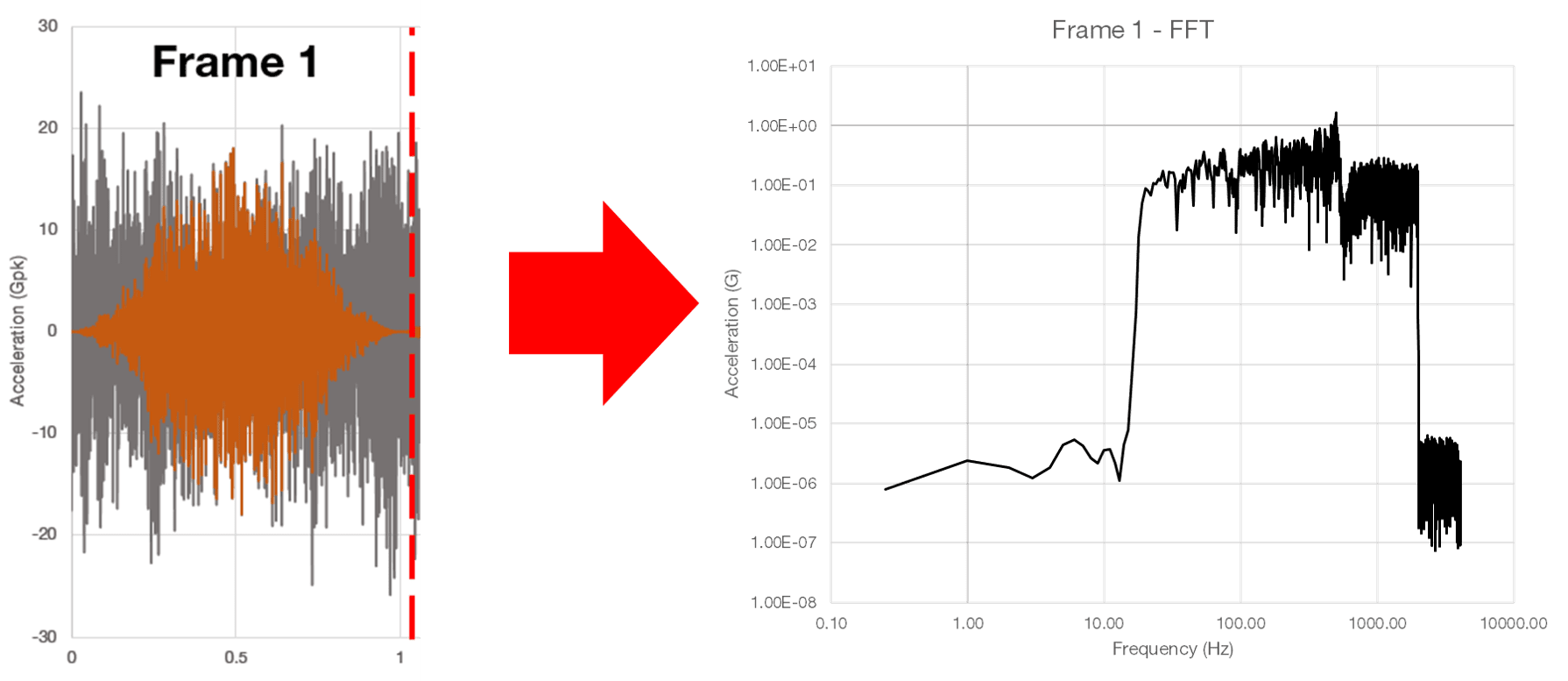Figure 1.4. Calculating the FFT for each frame.

FFT is used widely in signal analysis and processing. Most importantly, it shows the frequencies present in a waveform and in what proportions. This information can be used to determine many things: what frequencies are being excited during a section of time, the peak acceleration of each frequency inside of a windowed frame of data, the distribution of peaks, harmonic content, and more.

Certain weighting factors can also be applied to an FFT. If our goal was to simply generate an FFT, we might apply a weighting factor to ensure 1Gpk of the time domain data is equal to 1Gpk in the FFT. When the goal is to generate a PSD, the window function is normalized to preserve the input power.

3. Square the individual FFTs for each frame and find an average

Next, square the individual FFTs for each frame and then find the average squared-amplitude (Figure 1.5).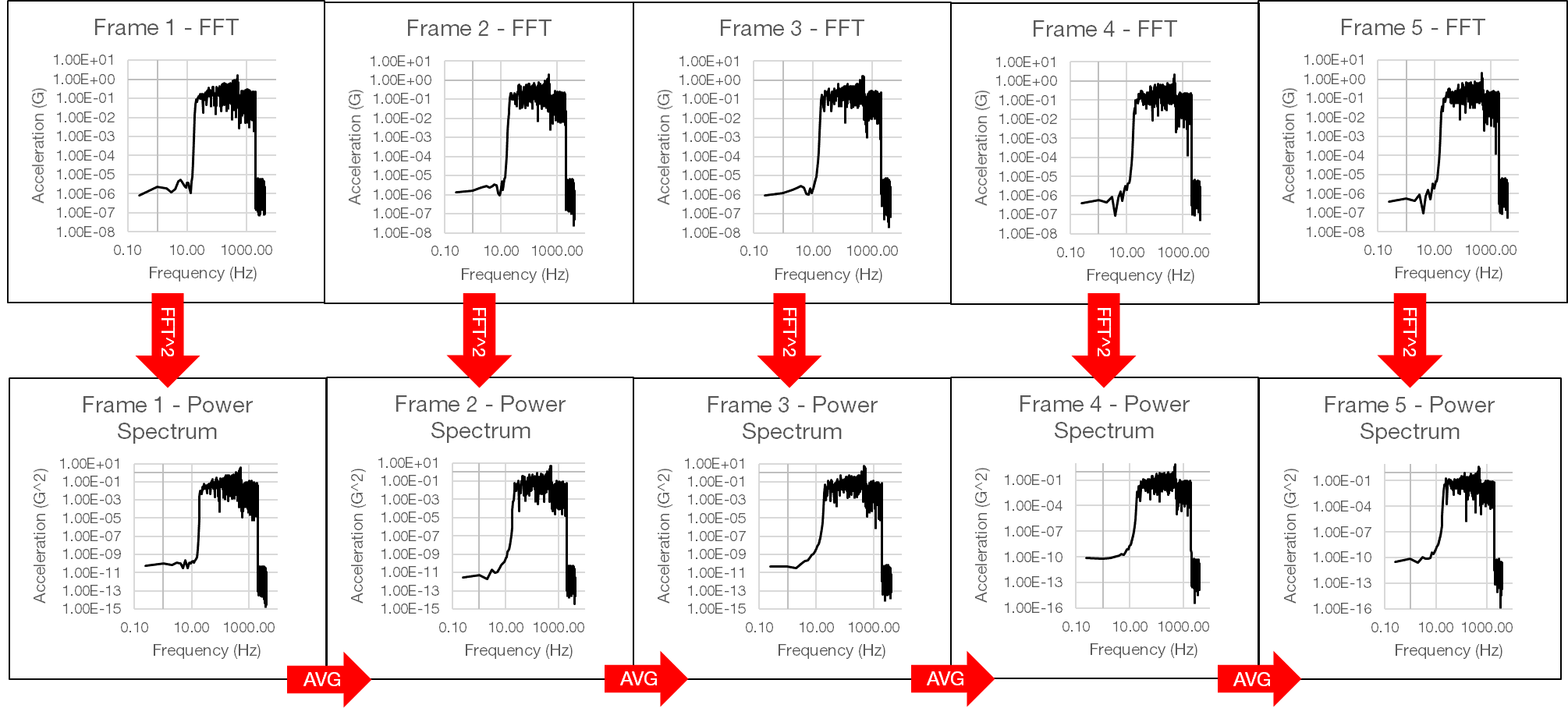Figure 1.5. Squaring and averaging FFTs.

The PSD shows the average energy at a single frequency over a period of time. Initially, it will have a variance or “hashiness.” As more frames of similar data are included in the average, the overall variance will decrease, the accuracy will increase, and the PSD will appear much smoother.

The total amount of time included in a PSD is related to the averaging parameter degrees of freedom (DOF). The more frames of data averaged together, the higher the DOF.  Simply put, more FFTs will result in better PSD. However, large numbers of FFTs require more time to collect and calculate.

4. Normalize the calculation to a single hertz

Finally, take the average squared FFT and divide it by the sample rate. By doing so, the data is normalized to a single hertz and a power spectral density is determined (Figure 1.6). For acceleration, the resulting unit is G2/Hz.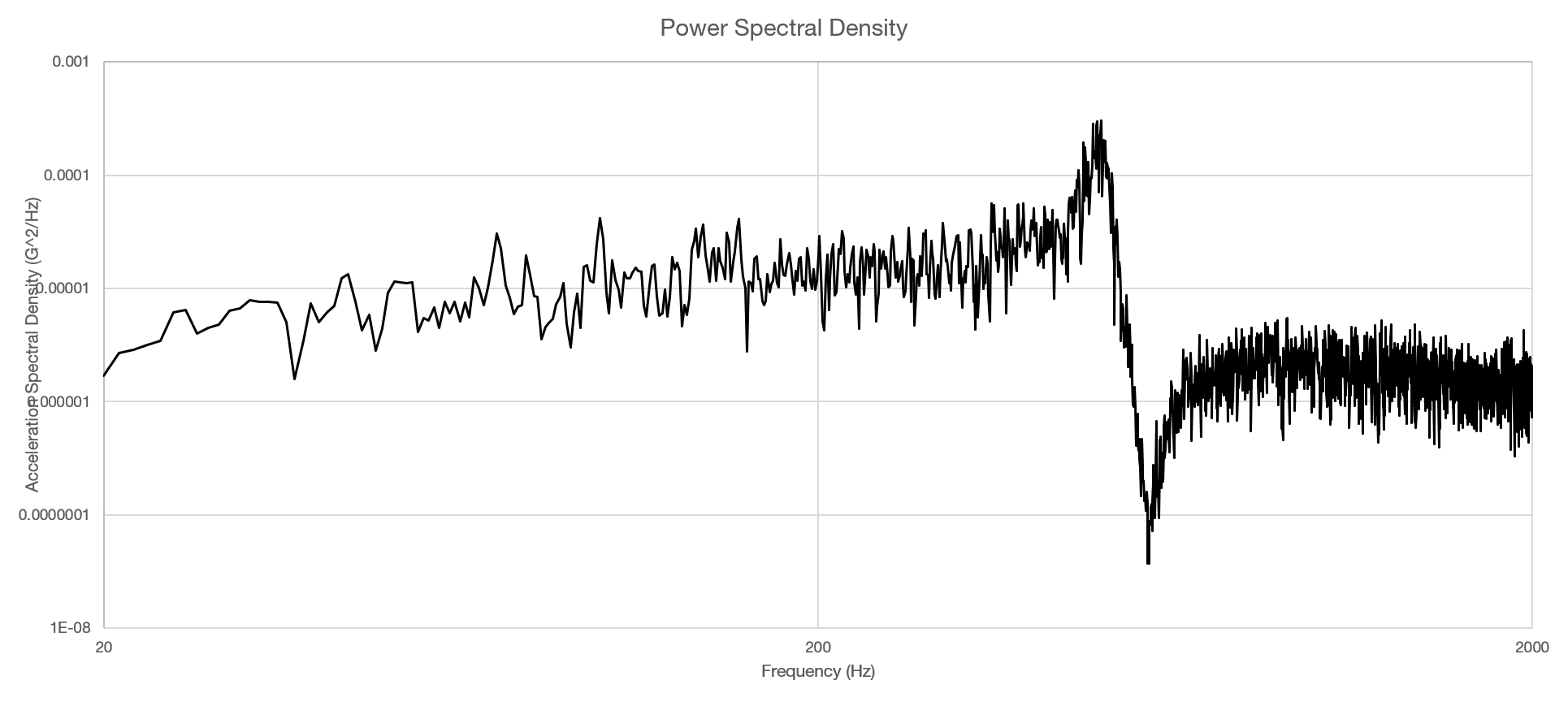Figure 1.6. The power spectral density (PSD).

Using the PSD, the response of the product under test is clear. As more frames of data are added to the PSD, the variance will continue to decrease and the PSD will become smoother.

For a more in-depth look at variance and methods of PSD smoothing, watch the webinar on Instant Degrees of Freedom, a patented feature from Vibration Research that quickly and effectively reduces the variance and creates a smooth PSD. This is the only mathematically-justified method for displaying a smooth PSD trace in a short period of time.

#### Overlapping

There is one additional technique that is often used, though not required, during PSD creation: overlapping. Overlapping is used to include more original data in the PSD and to generate more DOF for a period of time.

With a 0% overlap, each frame of data is completely separated. When frames are overlapped, some data in each frame is not accounted for due to the applied window functions. Additionally, for each frame of data included in the PSD, two DOFs are calculated for the total average.

For example, if we create a PSD with 0% overlap, 120 DOF, an 8,192Hz sample rate, 4,096 lines of resolution, and a Hanning window function (resulting in 1-second frames), we will need to average 60 seconds worth of data to achieve 120 degrees of freedom.

With a 50% overlap, there would be 0.5 seconds between the start of each frame (each frame would still be 1 second in length.) Frames do not result in 2 DOF per FFT when they are overlapped. A 50% overlap would result in around 1.85 DOF per FFT; a 75% overlap would result in around 1.2 DOF per FFT. Therefore, for a 50% overlap, 120 DOF, 8,192Hz, 4,096 lines of resolution, Hanning Window PSD, we can achieve the desired PSD in 64.8 frames in 32.4 seconds.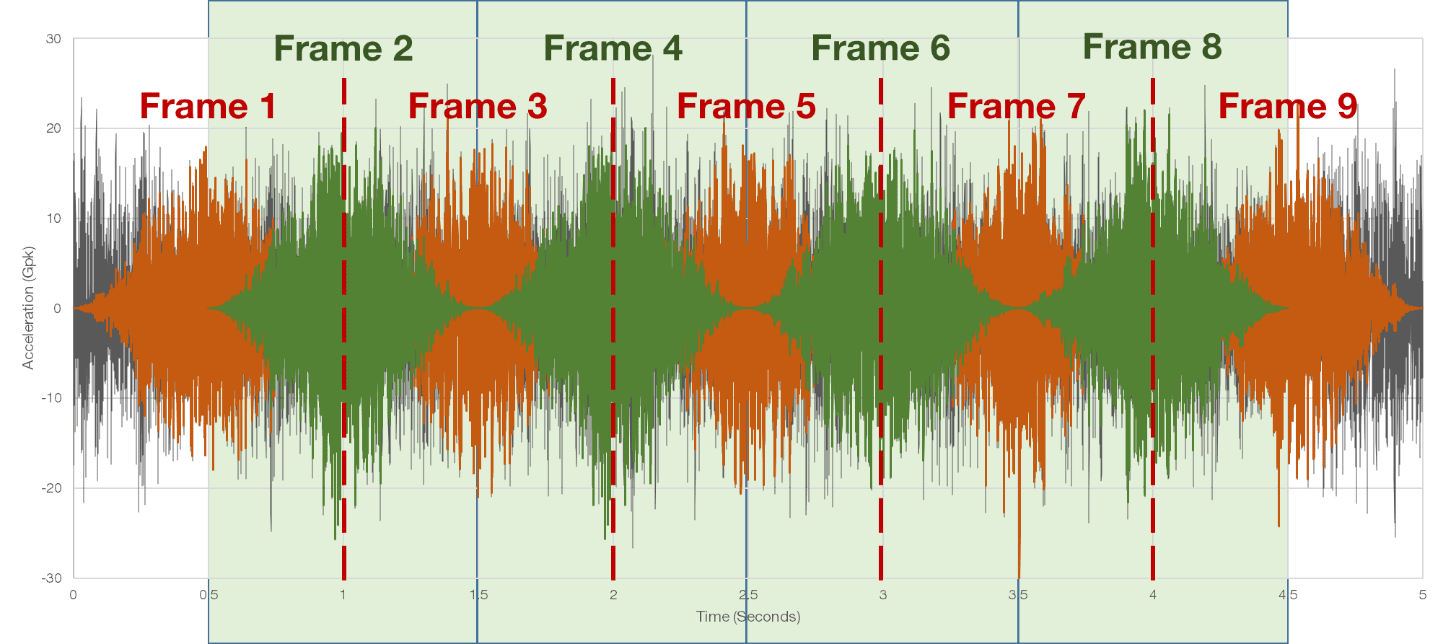Figure 1.7. Overlapping windowed frames.

In the original example with 0% overlap, there were five frames of data, resulting in 5 FFTs. With a 50% overlap, as shown in Figure 1.7, the same section of data results in 9 FFTs.

#### Lines of Resolution

The last parameter to consider in the PSD calculation is the lines of resolution. Lines of resolution, along with the sample rate, determine how far apart each analysis point is spaced on the PSD. A higher number of lines will result in a more accurate PSD but requires a larger number of samples to properly calculate.

Many test standards require a certain number of lines to be included inside a resonance to properly display the peak. If too few lines are used in a PSD, the result is similar to under-sampling a waveform: the distance between analysis points will be too great and the gap between not appropriately accounted for. A minimum requirement of three or more lines of resolution is needed to properly resolve a resonance.